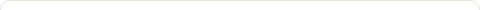Prime numbers using POSIX threads on Linux in [C]

Posted on: May 17, 2009

Prime Numbers

So, first lets talk a little about prime numbers. What makes a number prime? Well a prime number is prime when it is only divisible by one and itself. Take for example the number 3, 3 can only be divided by one and three and have a remainder of zero. If you try to divide by two then you will get a remainder of one. Therefore 3 is a prime number. Lets take another example the number 4, 4 is not a prime number because when you divide it by 2 you get a remainder of zero. Our definition states that a number can only have a remainder of zero when divided by one and itself, the number 4 clearly breaks this rule when divided by 2. 5 on the other hand can’t be divided by anything but one and itself so it’s prime. Get the hang of it yet?

So now that we understand what prime numbers are lets try and make a simple sudo code algorithm that depicts this behaviour. Lets say someone comes up to you on the street and asks you to list every prime number that comes before what ever number (we are going to call this the “upper limit”) they ask you.

FOR every number X that comes before our upper limit

FOR every number Y that comes before X

IF X MOD Y doesn’t equal zero

THEN this number must be prime

ELSE X must be divisible by some Y so it is NOT a prime number

[REMEMBER] Mod or Modulus (%) division is remainder division. EX 1: 3 %(MOD) 1 = 0; Because when you divide 3 by 1, 1 goes into 3, 3 times. This gives us a remainder of zero. EX 2: 5 % 3 = 2; Because 3 goes into 5 once, so 2 is left over as the remainder.

Modulus division is really going to help us with this prime number problem.

Okay, so you got the prime number thing down, you understand it (or will shortly 😉 ). Now it is time to talk about POSIX threads in linux. They kind of work like java threads in the sense that java has the run method it calls after you use the start function. Well, POSIX threads in linux have a create method that accepts a function as one of its parameters, this function is not called directly but rather the creation process calls it for you.

How do you create a thread?

Lets take a look at the code example below:

CODE:

#include <stdio.h>
#include <stdlib.h>

void *runner(void *param); /* the thread */

int main(int argc, char *argv[]) {

//Verify two args were passed in
if(argc < 2) {
fprintf(stderr, "USAGE: ./prime.out <Integer value>\n");
exit(1);
}

//verify the input is greater then or equal to two
if(atoi(argv) < 2) {
fprintf(stderr, "USAGE: %d must be >= 2\n", atoi(argv));
exit(1);
}

printf("Prime Numbers: ");

//Get the default attributes
//Wait for the thread to exit
printf("\nComplete\n");
}

//The thread will begin control in this function
void *runner(void *param) {
int i,j,upper = atoi(param);
/* Check to see if a number is prime */
for(i = 2; i < upper; i++) {
int trap = 0;
/* Check each number for divisibility */
for(j = 2; j < i; j++) {
int result = i % j;
/* If any of the numbers divide cleanly
then this number is not prime. So
stop checking. */
if(result == 0) {
trap = 1;
break;
}
}
//No numbers divided cleanly so this number must be prime
if(trap == 0) {
printf("[%d] ", i);
}
}
}

OUTPUT:

lee@isis:~\$ ./prime.out 100
Prime Numbers:                         

As you can see we made use of the POSIX pthread API. We set default attributes on the thread then created it. When we created it we passed in the thread ID, attributes, the function we need to call as the thread, and finaly the params. In this case we passed in the users input because it was already a pointer so it was easily passed with out casting.

In our prime number algorithm we don’t check 1 or the number itself to see if it divides cleanly, mainly because we already know it will so really there is no point in checking; it would also make our algorithim more complex for no reason and thats never a good thing.

Happy coding, hope this helped in some way. 🙂

[NOTE]: Compiling pthreads using gcc requires a command line option. EX. :gcc -lpthread -o prime.out prime.c

2 Responses to "Prime numbers using POSIX threads on Linux in [C]"[…] About Prime numbers using POSIX threads on Linux in [C] […][…] Prime numbers using POSIX threads on Linux in [C] […]